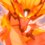# Trigamma Series

Evaluate $\sum_{k=1}^{\infty} \left[ \dfrac{\psi^{(1)} (k) }{k} \right]^{2}$

$\psi^{(1)} (k)$ is the trigamma function, defined as

$\psi^{(1)} (x) = \dfrac{\mathrm{d}^2}{\mathrm{d}x^2} [\ln (\Gamma (x) )]$

This is a part of the set Formidable Series and IntegralsNote by Ishan Singh
5 years, 5 months ago

This discussion board is a place to discuss our Daily Challenges and the math and science related to those challenges. Explanations are more than just a solution — they should explain the steps and thinking strategies that you used to obtain the solution. Comments should further the discussion of math and science.

When posting on Brilliant:

• Use the emojis to react to an explanation, whether you're congratulating a job well done , or just really confused .
• Ask specific questions about the challenge or the steps in somebody's explanation. Well-posed questions can add a lot to the discussion, but posting "I don't understand!" doesn't help anyone.
• Try to contribute something new to the discussion, whether it is an extension, generalization or other idea related to the challenge.

MarkdownAppears as
*italics* or _italics_ italics
**bold** or __bold__ bold
- bulleted- list
• bulleted
• list
1. numbered2. list
1. numbered
2. list
Note: you must add a full line of space before and after lists for them to show up correctly
paragraph 1paragraph 2

paragraph 1

paragraph 2

[example link](https://brilliant.org)example link
> This is a quote
This is a quote
    # I indented these lines
# 4 spaces, and now they show
# up as a code block.

print "hello world"
# I indented these lines
# 4 spaces, and now they show
# up as a code block.

print "hello world"
MathAppears as
Remember to wrap math in $$ ... $$ or $ ... $ to ensure proper formatting.
2 \times 3 $2 \times 3$
2^{34} $2^{34}$
a_{i-1} $a_{i-1}$
\frac{2}{3} $\frac{2}{3}$
\sqrt{2} $\sqrt{2}$
\sum_{i=1}^3 $\sum_{i=1}^3$
\sin \theta $\sin \theta$
\boxed{123} $\boxed{123}$

Sort by:

Here's a irrelevant comment:::: (Plagiarized Ishu's work)

Claim: $\displaystyle \sum_{k=1}^\infty ( \psi^{(1)} (k))^2 = 3 \zeta(3)$.

Proof:

By writing the trigamma function in terms of integral representation, we have $\displaystyle \psi^{(1)}(k) = \int_0^1 \dfrac{\ln x \cdot x^{k-1}}{1-x} \, dx$. Taking its square gives us

\begin{aligned} \displaystyle \left [ \psi^{(1)}(k) \right]^2 &= & \displaystyle \int_0^1\int_0^1 \dfrac{ \ln x \ln y (xy)^{k-1}}{(1-x)(1-y) } \, dx dy \\ \displaystyle \sum_{k=1}^\infty \left [ \psi^{(1)}(k) \right]^2 &= & \displaystyle \int_0^1\int_0^1 \dfrac{ \ln x \ln y}{(1-x)(1-y)} \sum_{k=1}^\infty (xy)^{k-1} \, dx dy \\ &= & \displaystyle \int_0^1 \int_0^1 \dfrac { \ln x \ln y}{(1-x)(1-y)(1-xy) } \, dx dy \\ &= & \displaystyle \int_0^1 \dfrac{ \ln x}{1-x} \int_0^1 \dfrac{ \ln y }{(1-y)(1-xy) } \, dy dx \end{aligned}

Consider the integral $\int_0^1 \dfrac{ \ln y }{(1-y)(1-xy) } \, dy = \displaystyle \dfrac y{y-1} \underbrace{\int_0^1 \dfrac {\ln y}{1-xy} \, dy}_{= \, A} - \dfrac1{y-1} \underbrace{\int_0^1 \dfrac{ \ln y}{y - 1} \, dy}_{= \, B}$

Solving for $A$ gives

\begin{aligned} \displaystyle \int_0^1 \dfrac {\ln y}{1-xy} \, dy &=& \displaystyle \int_0^1 \ln y \sum_{x=1}^\infty (xy)^{n-1} \, dy \\ &=& \displaystyle \sum_{n=1}^\infty x^{n-1} \int_0^1 \ln y \cdot y^{n-1} \, dy \\ &=& - \displaystyle \sum_{n=1}^\infty \dfrac{x^{n-1}}{n^2} = -\dfrac{ \text{Li}_2 (x)} x \end{aligned}

Similarly, solving for $B$ gives $- \dfrac{\text{Li}_2 (1) }1 = - \dfrac{\pi^2}6$.

Thus we have $\displaystyle \int_0^1 \dfrac{ \ln y}{(1-y)(1-xy) } \, dy = \dfrac1{1-x} \left [ \text{Li}_2 (x) - \dfrac{\pi^2} 6 \right ]$, and so the double integral becomes

$\int_0^1 \dfrac {\ln x}{(1-x)^2 } \left [ \text{Li}_2 (x) - \dfrac{\pi^2} 6 \right ] \, dx = \int_0^1 \dfrac {\ln (1-x)}{x^2 } \left [ \text{Li}_2 (1-x) - \dfrac{\pi^2} 6 \right ] \, dx$

Because $\displaystyle \int_a^b f(x) \, dx = \int_a^b f(a+b-x) \, dx$. We integrate by parts with $du = \dfrac{ \ln(1-x)}{x^2}, v = \text{Li}_2 (x) - \dfrac{\pi^2} 6$ to get

$u = \int_0^x \dfrac{\ln (1-t)}{t^2} \, dt = -\dfrac{(1-x)}x - \int_0^x \dfrac {dt}{t(1-t)} = \ln(1-x) - \ln(x) - \dfrac{ \ln(1-x)}x$

And $\dfrac{dv}{dx} = \left [ \text{Li}_2 (x) - \dfrac{\pi^2} 6 \right ] = \dfrac{\ln x}{1-x}$.

Integrate by parts gives us

\begin{aligned} \displaystyle \int_0^1 \dfrac {\ln (1-x)}{x^2 } \left [ \text{Li}_2 (1-x) - \dfrac{\pi^2} 6 \right ] \, dx &=& - \displaystyle \int_0^1 \dfrac{ \ln x}{1-x} \left [ \ln(1-x) - \ln x - \dfrac{ \ln(1-x)}x \right ] \, dx \\ &=& -\displaystyle \underbrace{ \int_0^1 \dfrac{ \ln x \ln(1-x)}{1-x} \, dx}_{= \, \rm{I}} + \underbrace{ \int_0^1 \dfrac{ (\ln x)^2}{1-x} \, dx}_{= \, \rm{II}}+ \underbrace{ \int_0^1 \dfrac{ \ln x \ln(1-x)}{x(1-x)} \, dx}_{= \, \rm{III}} \end{aligned}

Now we just need to find the values of $\rm{I}, \rm{II}$ and $\rm{III}$. Taking note that $B(a,b)$ is the beta function, we have

\begin{aligned} \displaystyle \rm{I} &=& \displaystyle\int_0^1 \dfrac{ \ln x \ln(1-x)}{1-x} \, dx \\ \displaystyle &=& \displaystyle \lim_{a\to0^+}\lim_{b\to1} \dfrac {\delta}{\delta a} \left( \dfrac {\delta}{\delta b} B(a,b) \right) = \zeta(3) \\ \displaystyle \rm{II} &=& \displaystyle\int_0^1 \dfrac{ (\ln x)^2}{1-x} \, dx \\ \displaystyle &=& \displaystyle \lim_{a\to0^+}\lim_{b\to1} \dfrac {\delta^2}{\delta a^2} \left ( B(a,b) \right) = 2\zeta(3) \\ \displaystyle \rm{III} &=& \displaystyle\int_0^1 \dfrac{ \ln x \ln(1-x)}{x(1-x)} \, dx \\ \displaystyle &=& \displaystyle \lim_{a\to0^+}\lim_{b\to0^+} \dfrac {\delta}{\delta a} \left( \dfrac {\delta}{\delta b} B(a,b) \right) = 2\zeta(3) \end{aligned}

Combining them all together:

$\displaystyle - \int_0^1 \dfrac {\ln (1-x)}{x^2 } \left [ \text{Li}_2 (1-x) - \dfrac{\pi^2} 6 \right ] \, dx =- \zeta(3) + 2\zeta(3) + 2\zeta(3) = \boxed{3\zeta(3)} .$

- 5 years, 5 months ago

Nice one! It doesn't matter if it is plagiarised or not.

- 5 years, 5 months ago

Try solving the actual question! =D

- 5 years, 5 months ago

$\displaystyle \frac{93}{24}\zeta(6)$

- 5 years, 4 months ago

That's incorrect.

- 4 years, 1 month ago

Hi! Looks like a calculation error (oops! the sum should also contain some $\zeta(3)^2$ term). Anyway, it was solved here http://integralsandseries.prophpbb.com/topic681.html

- 4 years, 1 month ago

Nice. I have discovered another solution which allows us to generalize the result to higher powers and other polygamma functions as well.

- 4 years, 1 month ago